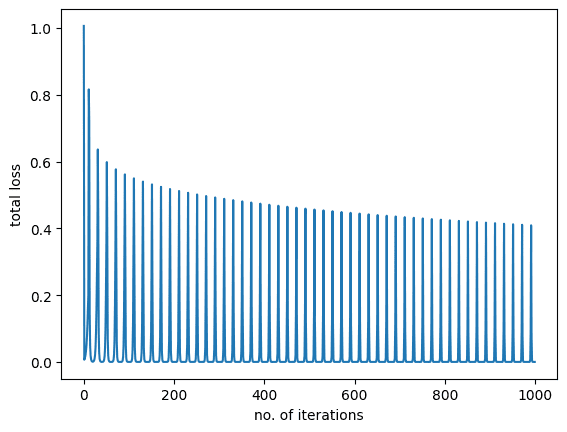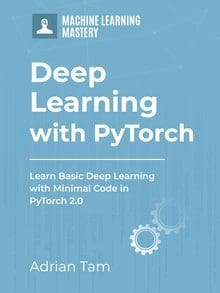# Training Logistic Regression with Cross-Entropy Loss in PyTorch

Last Updated on April 8, 2023

In the previous session of our PyTorch series, we demonstrated how badly initialized weights can impact the accuracy of a classification model when mean square error (MSE) loss is used. We noticed that the model didn’t converge during training and its accuracy was also significantly reduced.

In the following, you will see what happens if you randomly initialize the weights and use cross-entropy as loss function for model training. This loss function fits logistic regression and other categorical classification problems better. Therefore, cross-entropy loss is used for most of the classification problems today.

In this tutorial, you will train a logistic regression model using cross-entropy loss and make predictions on test data. Particularly, you will learn:

• How to train a logistic regression model with Cross-Entropy loss in Pytorch.
• How Cross-Entropy loss can influence the model accuracy.

Kick-start your project with my book Deep Learning with PyTorch. It provides self-study tutorials with working code.

Let’s get started.Training Logistic Regression with Cross-Entropy Loss in PyTorch.
Picture by Y K. Some rights reserved.

## Overview

This tutorial is in three parts; they are

• Preparing the Data and Building a Model
• Model Training with Cross-Entropy
• Verifying with Test Data

## Preparing the Data and the Model

Just like the previous tutorials, you will build a class to get the dataset to perform the experiments. This dataset will be split into train and test samples. The test samples are an unseen data used to measure the performance of the trained model.

First, we make a Dataset class:

Then, instantiate the dataset object.

Next, you’ll build a custom module for our logistic regression model. It will be based on the attributes and methods from PyTorch’s nn.Module. This package allows us to build sophisticated custom modules for our deep learning models and makes the overall process a lot easier.

The module consist of only one linear layer, as follows:

Let’s create the model object.

This model should have randomized weights. You can check this by printing its states:

You may see:

### Want to Get Started With Deep Learning with PyTorch?

Take my free email crash course now (with sample code).

Click to sign-up and also get a free PDF Ebook version of the course.

## Model Training with Cross-Entropy

Recall that this model didn’t converge when you used these parameter values with MSE loss in the previous tutorial. Let’s see what happens when cross-entropy loss is used.

Since you are performing logistic regression with one output, it is a classification problem with two classes. In other words, it is a binary classification problem and hence we are using binary cross-entropy. You set up the optimizer and the loss function as follows.

Next, we prepare a DataLoader and train the model for 50 epochs.

The output during training would be like the following:

As you can see, the loss reduces during the training and converges to a minimum. Let’s also plot the training graph.

You shall see the following:## Verifying with Test Data

The plot above shows that the model learned well on the training data. Lastly, let’s check how the model performs on unseen data.

which gives

When the model is trained on MSE loss, it didn’t do well. It was around 57% accurate previously. But here, we get a perfect prediction. Partially because the model is simple, a one-variable logsitic function. Partially because we set up the training correctly. Hence the cross-entropy loss significantly improves the model accuracy over MSE loss as we demonstrated in our experiments.

Putting everything together, the following is the complete code:

## Summary

In this tutorial, you learned how cross-entropy loss can influence the performance of a classification model. Particularly, you learned:

• How to train a logistic regression model with cross-entropy loss in Pytorch.
• How Cross-Entropy loss can influence the model accuracy.

## Get Started on Deep Learning with PyTorch!#### Learn how to build deep learning models

...using the newly released PyTorch 2.0 library

Discover how in my new Ebook:
Deep Learning with PyTorch

It provides self-study tutorials with hundreds of working code to turn you from a novice to expert. It equips you with
tensor operation, training, evaluation, hyperparameter optimization, and much more...

### One Response to Training Logistic Regression with Cross-Entropy Loss in PyTorch

1.Michael March 28, 2023 at 7:31 am #

Hi M:

Nice code, though I believe you need to perform a training/test split of the data to demonstrate the model is actually generalising well.